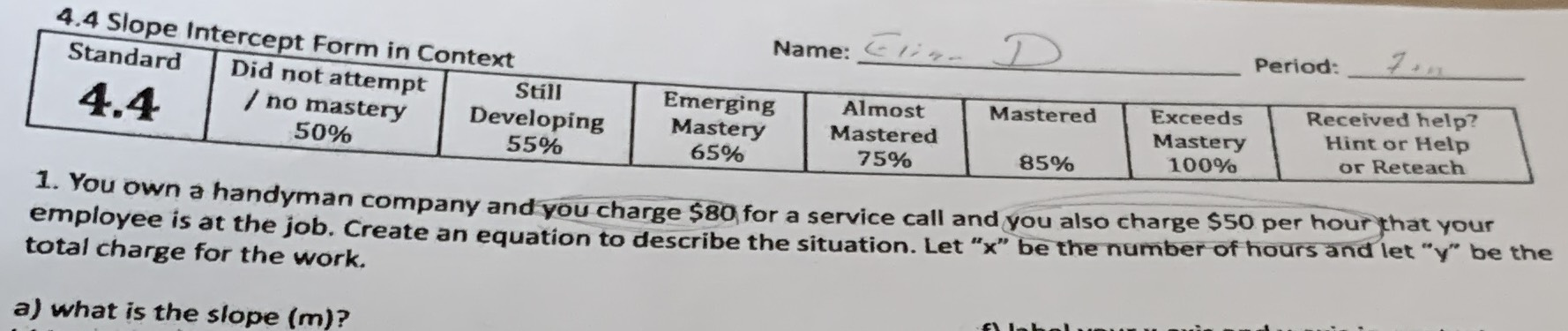### ¿Todavía tienes preguntas de matemáticas?

Pregunte a nuestros tutores expertos
Algebra
Pregunta1. You own a handyman company and you charge $$\ 80$$ for a service call and you also charge $$\ 50$$ per hour that your employee is at the job. Create an equation to describe the situation. Let " $$x$$ " be the number of hours and let "y" be the total charge for the work. a) what is the slope $$( m )$$ ?